#Quality Digest

Featured Product
This Week in Quality Digest Live
Statistics Features
Donald J. Wheeler
What do predictable processes have in common with chaos?
William A. Levinson
People can draw the wrong conclusions due to survivor, survey, and bad news bias.
Donald J. Wheeler
Does your approach do what you need?
Paul Laughlin
Correlation vs. causality
Donald J. Wheeler
In spite of what everyone says to the contrary
Statistics News
New capability delivers deeper productivity insights to help manufacturers meet labor challenges
Day and a half workshop to learn, retain, and transfer GD&T knowledge across an organization
Elsmar Cove is a leading forum for quality and standards compliance
InfinityQS’ quality solutions have helped cold food and beverage manufacturers around the world optimize quality and safety
User friendly graphical user interface makes the R-based statistical engine easily accessible to anyone
Collect measurements, visual defect information, simple Go/No-Go situations from any online device
Good quality is adding an average of 11 percent to organizations’ revenue growth
Ability to subscribe with single-user minimum, floating license, and no long-term commitment
A guide for practitioners and managersBio

Statistics

## The Ability to Detect Signals

### Process behavior charts and skewed data

Published: Monday, October 7, 2019 - 12:03

Last month I looked at how the fixed-width limits of a process behavior chart filter out virtually all of the routine variation regardless of the shape of the histogram. In this column I will look at how effectively these fixed-width limits detect signals of economic importance when skewed probability models are used to compute the power function.

### Power functions

A power function provides a mathematical model for the ability of a statistical procedure to detect signals. Here we shall use power functions to define the theoretical probabilities that an X chart will detect different sized shifts in the process average. To compute a power function we begin with a probability model to use, and a shift in location for that model. Figure 1 shows these elements for a traditional standard normal probability model.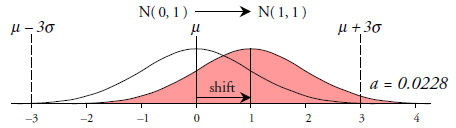Figure 1: Normal model with a 1.0-sigma shift in location

The probability that a point will fall above the upper three-sigma limit when the process mean has shifted from 0.0 to 1.0 is α = 0.0228. This is the probability of detecting this shift on the first observation following the shift (k = 1). The probability of detecting the shift when k = 2 is:And the sum of these two values is the probability of detecting this shift within two observations. This sum of 0.0451 is the “power for detecting a one-sigma shift” at k = 2. Continuing in this manner, the probability that a point will fall outside a three-sigma limit within k observations is: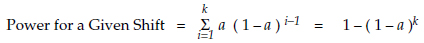Thus, our initial probability of a point falling outside the three-sigma limit, α, depends upon a probability model and the size of the shift. When combined with k = the number of observations following the shift the power function can be evaluated using the simple formula above. When we compute these probabilities for different shifts and different values for k we can draw the power function curves for the X chart shown in figure 2.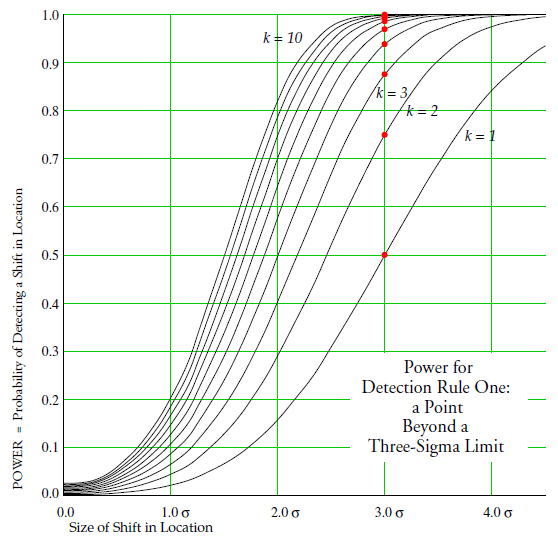Figure 2: The traditional power function curves for an X chart

To interpret figure 1 consider the red dots shown which correspond to a 3σ shift in location. There is a 50-percent chance of detecting this shift on the very first observation following the shift. There is a 75-percent chance of detecting this shift within two observations, and there is an 87.5-percent chance of detecting this shift within three observations following the shift. Thus, by covering different sized shifts and different numbers of observations, the curves in figure 2 contain a wealth of information. To summarize this information in a coherent and understandable way we shall use average run lengths.

### Average run lengths

Returning to the red dots in figure 2 where k = 1, 2, 3, 4, 5, etc. We could compute the average value for k needed to detect a 3σ shift in location. This average is known as the average run length (ARL) and may be computed by multiplying each value of k by the probability of detecting the shift on the k-th observation, and then adding up the products. For the red dots this operation gives: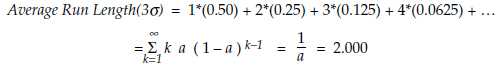So an X chart is traditionally said to have an ARL of 2.0 for detecting a 3σ shift. This means that, on the average, the chart will detect a signal of this size within two observations. Thus, an ARL value summarizes the ability to detect a specific shift.

Since the probability α can never exceed 1.00, the ARL values can never be less than 1.00. By using the ARL values for different sized shifts we can summarize a set of power function curves quite compactly.Figure 3: Traditional average run lengths for an X chart

As expected, as the shifts get bigger the ARL values get smaller, and the larger shifts are detected more quickly. In what follows I shall use the ARL values to evaluate the ability of an X chart to detect signals while using skewed probability models.

### The six probability models

The curves shown in figure 2 are the traditional power functions based on the normal probability model. But what happens to the ability of the X chart to detect signals when using a skewed probability model? To answer this question I computed the power function curves for the X chart using the five skewed probability models shown in figure 4.

The chi-square distribution with 8 degrees of freedom has a mean value that is 2.0 standard deviations above zero.

The Weibull distribution with shape parameter = 1.6 has a mean value that is 1.56 standard deviations above zero.

The chi-square distribution with 4 degrees of freedom has a mean value that is 1.414 standard deviations above zero.

The exponential distribution has a mean value that is 1.00 standard deviation above zero.

The lognormal distribution with shape parameter = 1.00 has a mean value that is 0.76 standard deviations above zero.

I computed the power functions for each of these six probability models using five different combinations of the Western Electric zone tests. However, it turns out that using the various run-tests in addition to detection rule one will add very little to the power functions for the skewed probability models. So, in the interest of simplicity, I shall only consider the power functions for detection rule one (a single point beyond a three-sigma limit) in the evaluations that follow.Figure 4: Six probability models

As always, when a boundary condition falls inside one of the three-sigma limits it will take precedence over that limit, and the process behavior chart will become a one-sided chart as shown. It is instructive to note that, in every case, the upper three-sigma limits continue to cover the bulk of the elongated tails in spite of the increasing skewness.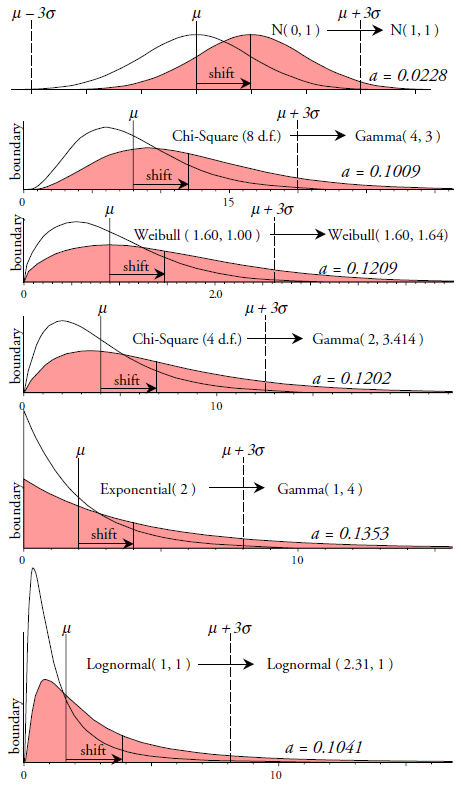Figure 5: The six models with one-sigma shifts

Figure 5 shows the original distributions and the distributions used to represent a one-sigma shift in location. With the skewed probability models any change in location will generally be accompanied by a change in dispersion. To maintain the same amount of skewness in spite of the change in both location and dispersion I had to use gamma distributions to represent the shifted chi-square and exponential distributions. Since gamma distributions possess both a scale parameter and a shape parameter their use allowed the average to shift while maintaining the skewness of the original distributions. Inverting the values for the probabilities of exceeding the upper three-sigma limit for each of the six models (labeled α in figure 5) results in the ARL values in the first row of figure 7.

### The results

Process behavior charts are intended to detect those process changes that are large enough to be of economic interest. In most cases these will be shifts in location in the neighborhood of three sigma or greater. Figures 6 and 7 show the ARL curves for the six different probability models for shifts greater than 2σ. While all of these curves drop as we move to the right, the ARL values increase as the skewness of the model increases. Thus these different ARL curves quantify the differences in sensitivity that occur as the probability model becomes more skewed.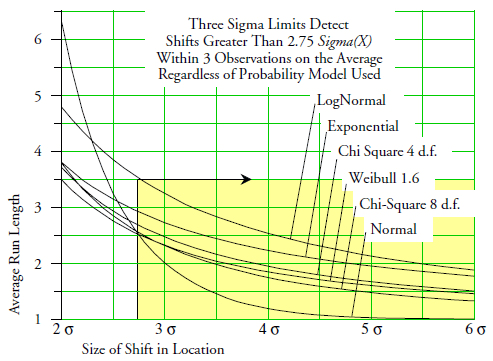Figure 6: Average run length (ARL) curves for detection rule one

In the region where the normal model has the smallest ARL value we find the following from figure 7: For a 2.8σ shift in location the ARL value moves up from 2.4 to 2.5, 2.5, 2.6, 2.9, and 3.5 as the probability model changes. For a 3σ shift in location the ARL value moves up from 2.0 to 2.3, 2.3, 2.5, 2.7, and 3.2. So we can expect that shifts in the neighborhood of 3σ to be detected within 2 to 3 observations on the average regardless of which of these six probability models we use to define the power function.

For a 4σ shift in location the ARL value moves up from 1.2 to 1.7, 1.8, 1.9, 2.2, and 2.5. For a 5σ shift in location the ARL value moves up from 1.0 to 1.5, 1.6, 1.7, 1.9, and 2.1. And for a 6σ shift in location the ARL value moves up from 1.0 to 1.3, 1.4, 1.5, 1.8, and 1.9. So we can expect shifts of 4σ to 6σ to be detected within 1 to 2 observations on the average regardless of which of these six probability models we use to define the power function.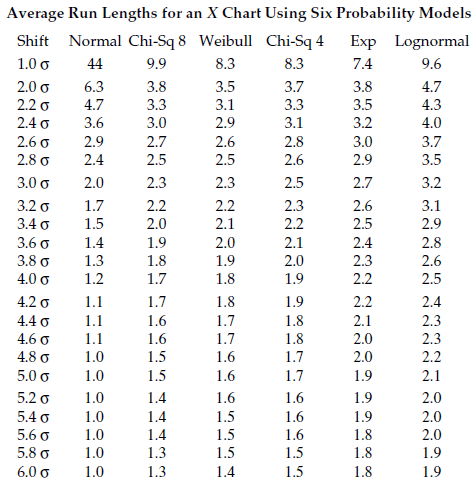Figure 7: Average run lengths for an X chart under six probability models

Thus, these ARL values tell us that with the generic, three-sigma limits, depending upon which probability model you think is appropriate, you might have to wait, on the average, for one extra observation to detect these signals!

Unfortunately, in practice, we will never have enough data to actually choose between these various probability models. This means that we will never be able to identify which ARL curve above approximates our analysis. But because these ARL values are all so similar, we can definitely say that by the time we are looking at signals greater than 2.75σ, all of the probability models have a theoretical average run length below 3.5. This means that in practice an X chart will usually detect shifts in excess of 2.75σ within an average of three observations or less. Moreover, shifts in excess of 4σ will usually be detected within an average of one or two observations.

So different probability models do result in different power functions. We have rigorously quantified these differences across a wide range of skewed probability models and have found that, for shifts in location that are large enough to be of interest, the theoretical differences are all too small to be of any practical consequence.

### Skewed histograms

Of course, the most common cause of a skewed histogram is not a skewed process, but rather a process that is operated unpredictably. As the process location goes on walkabout the outcomes vary and the group picture turns out to be lopsided. Consider the histogram in figure 8. It could hardly be said to be anything other than skewed.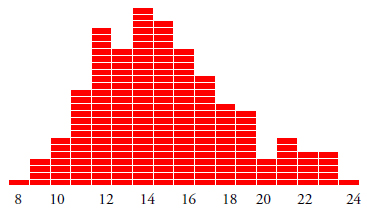Figure 8: Histogram for 200 process values

When we place these 200 values on an X chart in time-order sequence with limits based on the average moving range of 2.38 we get figure 9. With 12 points outside the limits we have plenty of signals. This process was changing during the time covered by these data and any attempt to discuss the “skewness” of the histogram above, or to fit a probability model to these data, is patent nonsense.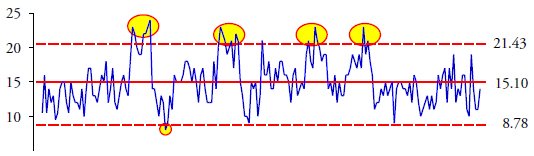Figure 9: X chart for figure 8 data

We cannot use a probability model to describe a process that is changing. But how can you know if the process is changing? That is the purpose of a process behavior chart. So trying to fit a probability model to your data before you place them on a process behavior chart does not make sense. Never has, never will.

### Predictable processes

On the other hand, when a process is operated predictably and the process average is close to some barrier or boundary condition we will end up with a skewed histogram. As the distance between the process average and the boundary condition drops below two standard deviations the skewness will become more pronounced and the histogram will display one short tail and one long tail. So do we need to fit a model to these data so that we can fine-tune the limits to make the process behavior chart more sensitive? No, we do not. Why we do not will be explained in next month’s column.

### Summary

Probability theory only provides a guide for practice. To compute power functions we have to assume that:
1. The measurements do not display any discreteness
2. The measurements are independently and identically distributed
3. We know the probability model for the measurements
4. The limits are known without error
5. Any changes in process location can be represented by a step function.

While these assumptions make the computations possible, they all, to a greater of lesser degree, are unrealistic in practice. This is why power functions are said to be theoretical. They only approximate what happens when we analyze data. When theoretical values turn out to be similar, the theoretical differences are unlikely to be realized in practice.

After a careful and rigorous theoretical analysis that is sufficiently general to cover most situations we have found that skewness might slow the detection of shifts in location that are 3σ and larger by an average of one additional observation when using generic, three-sigma limits. Since ARL differences of this size will be undetectable in practice, we must conclude that skewness is not a problem for a process behavior chart.

Do not try to fit a probability model to your data.

And do not even think about using transformations to achieve “normality.”

Simply collect the data, place them on a process behavior chart, and determine if your process is being operated predictably or unpredictably. Look for assignable causes of unpredictable operation and remove their effects from your process. Repeat. In doing this you can make a process behavior chart into the locomotive of continual improvement. Everything else is just unnecessary busywork.

### Discuss### Donald J. Wheeler

Find out about Dr. Wheeler’s virtual seminars for 2022 at www.spcpress.com. Dr. Wheeler is a fellow of both the American Statistical Association and the American Society for Quality who has taught more than 1,000 seminars in 17 countries on six continents. He welcomes your questions; you can contact him at djwheeler@spcpress.com.